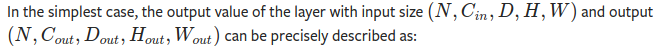# How to understand conv3d input and oupout?

Hello,

##How to understand the D ? in (N, C, D, H, W)?
let’s say for example I have five video frames and I stack the frames along the channel dimension giving me :
a (1, 15, H, W) tensor assuming RGB frames. How do I reshape this tensor to (N, C, D, H, W)

N is the batch size.
H and W are the height and width of the video frame.
Now C and D. I am not sure about which one is the 3 for RGB and 5 for video frames.
I guess 5 would the channels, C=5, and D=3 for the dimension of each video frame.
You cant predefined the batch size in getitem().
try to debug with batch size = 1.

@ptrblck could you please explain this ? the documentation does not explain the D in (N, C, D, H, W).

The `D` dimension should define the “depth” of a volume for 3D inputs or parameters.
While 2-dimensional data defines spatial dimensions as height and width, 3-dimensional data uses depth, height, and width.

For my example above, Given a Tensor (N, 15, H, W) in 2d. Its 3D equivalent is : (N, 3, 5, H, W) where 3 is the number of channels for RGB and 5 the number of frames.